# Cell Arrays in Julia

Cell array is an abstract data type with indexed data containers called cells, where each cell can contain any type of data. It is normally used in Matlab to store data.

Coming to Julia, one of the magical things about Julia is its type system. It is a strictly typed language. In Julia, arrays can contain values of homogeneous [1, 2, 3] or heterogeneous types [1, 2.5, “3”]. Julia will try to promote the values to a common concrete type by default. If Julia can not promote the types contained, the resulting array would be of the abstract type Any.

Examples:

 `# Creating an Array of type Int  ` `[``1``, ``2``, ``3``] ` ` `  `# Creating an Array of type Float ` `[``1``, ``2.5``, ``3``] ` ` `  `# Creating an Array of type Any ` `[``1``, ``2.3``, ``"3"``] `

Output: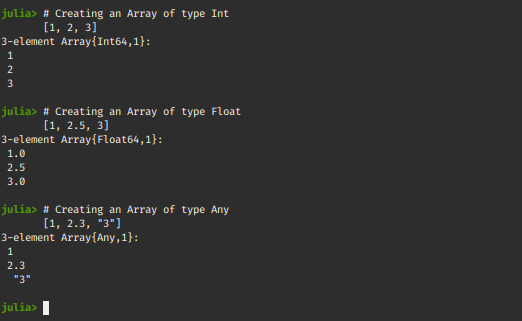So it can be said that an Array{Any} is equivalent to a Matlab cell array. It can hold elements of different Data Types.

#### Creating a cell array from a regular array

A cell array can be created with a regular array with the use of some pre-defined methods in Julia.
Let’s create an Array of type Int and see it’s conversion from a regular Array to a Cell Array!

 `# Creating an Array of type Int ` `a ``=` `[``1``, ``2``, ``3``] ` `     `  `# push an Int into the Array ` `push!(a, ``5``) ` `  `  `# So the type Int is retained ` ` `  `# Push a Float value into the Array ` `push!(a, ``5.2``) `

Output: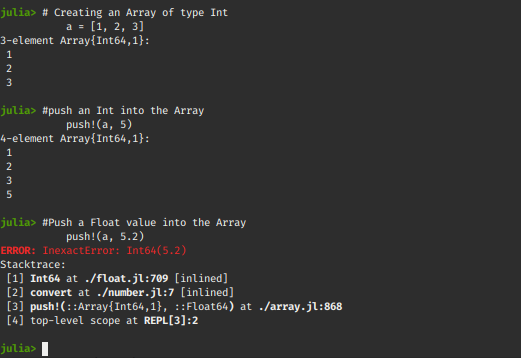Above code generates an error when we try to push a float value in an Integer typed Array. This shows that Julia is strictly typed and doesn’t allow pushing elements of any other data type.

Changing Data type of an Array: Julia by default doesn’t change the type of an Array and hence it doesn’t allow pushing differently typed value in the array. This can be avoided with the use of external packages.

Example:

 `# A special package to allow extended operations with push!() ` `# Install it by typing "add BangBang" ` `using BangBang ` ` `  `# Creating an Array of type Int ` `a ``=` `[``1``, ``2``, ``3``] ` `     `  `# push an Int into the Array ` `push!(a, ``5``) ` ` `  `# Let's try to push the Float value now ` `push!!(a, ``5.2``) `

Output: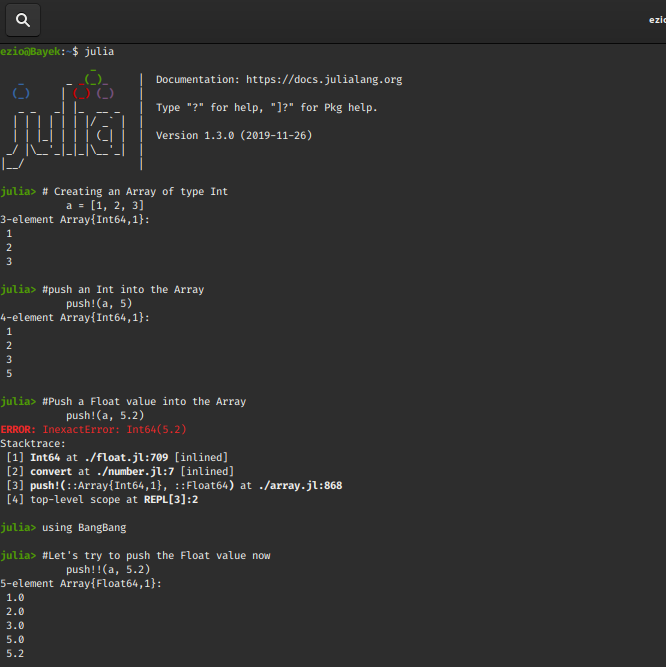Above code converts the Array to Float64, similarly, it can be used to convert the same to a string array, etc.

Example:

 `using BangBang ` ` `  `# Creating an Array of type Int ` `a ``=` `[``1``, ``2``, ``3``] ` `     `  `# push an Int into the Array ` `push!(a, ``5``) ` ` `  `# Pushing the Float value ` `push!!(a, ``5.2``) ` ` `  `# Let's try to store a different data type now ` `push!!(a, ``"GeeksForGeeks"``) `

Output: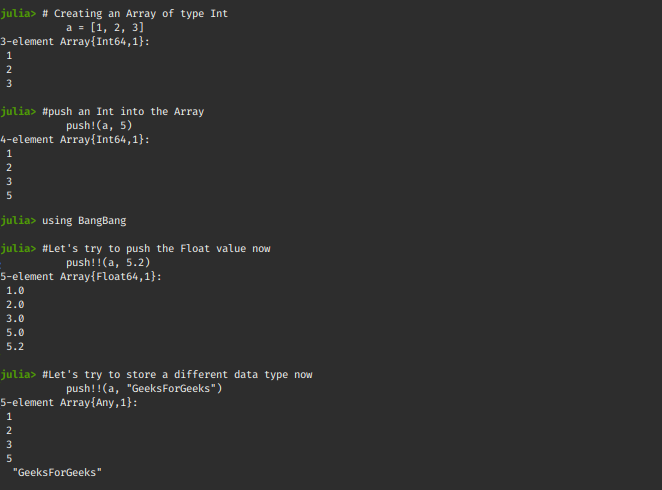This converts a regular Array into a cell Array which holds values of heterogeneous data types.

Cell Arrays of 2D Arrays:

 `# Create a 2x2 Array of type Int ` `[``1` `2` `; ``3` `4``] ` `    `  `# Create a 2x2 Array of type Float ` `[``1` `2` `; ``3` `4.2``]  ` ` `  `# Create a 2x2 Cell Array, of type Any ` `[``1` `2` `; ``3` `"foo"``] `

Output: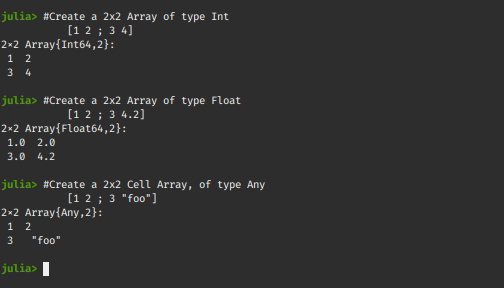Cell Arrays of 3D array:

 `# Create a 2x2x2 Array of type Int ` `cat([``1` `2``; ``3` `4``], [``5` `6``; ``7` `8``], dims``=``3``) ` `    `  `# Create a 2x2x2 Cell Array, of type Any ` `cat([``1` `"foo"``; ``3.2` `4``], [``5` `"baz"``; ``7` `8``], dims``=``3``) `

Output: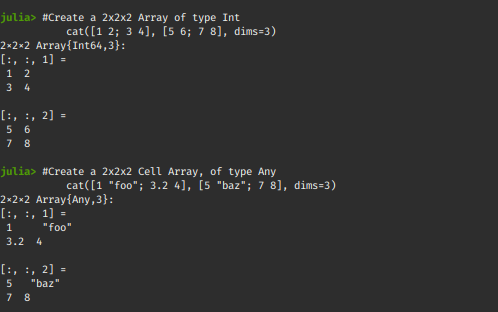#### Adding rows to a Cell Array

Additional rows can be added to the end of the cell array with the use of push!() function.

 `# Create 3 element Cell Array,  ` `# that contains sub-arrays of type Any ` `c ``=` `[[``10` `20` `"GeeksForGeeks"``], [``40.0``], [``50` `60``]] ` `    `  `# push a row Cell Array, of type Any ` `c ``=` `push!(c, [``1` `2` `10``]) `

Output: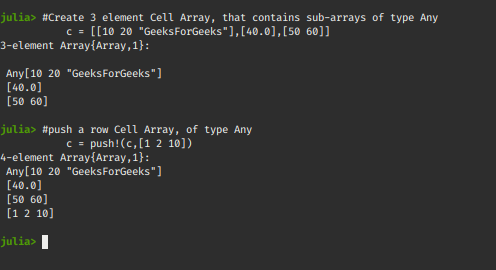Storing Cell Arrays within Cell Arrays:

 `# Create two, 3 element Cell Arrays i and j ` `i ``=` `[``2``, ``3.0``, ``"GeeksForGeeks"``] ` `j ``=` `[``2.3``, ``3.3``, ``"CellArrays"``] ` `     `  `# Create a 3x2 Array ` `IJ ``=` `[i j] `

Output: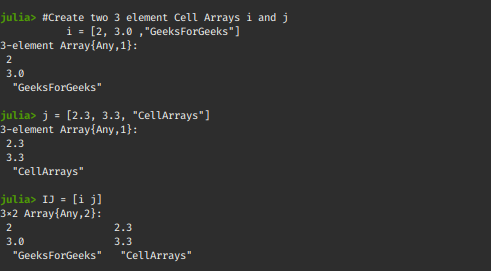#### Use of Cell Arrays

A cell array is just like a regular Julia array. They are used across various applications, as the representation of DataFrames, Tuples, etc.

Cell Arrays in Julia are also used to store input arguments for a function.

 `# Create two Arrays ` `i ``=` `[``2``, ``3``, ``4``] ` `j ``=` `[``2.3``, ``3.3``, ``4.3``] ` ` `  `# A function that uses values  ` `# from the above two arrays ` `function FOO(i, j) ` `    ``a ``=` `i ``+` `j ` `end ` `     `  `# Calling the function ` `FOO(i, j) `

Output: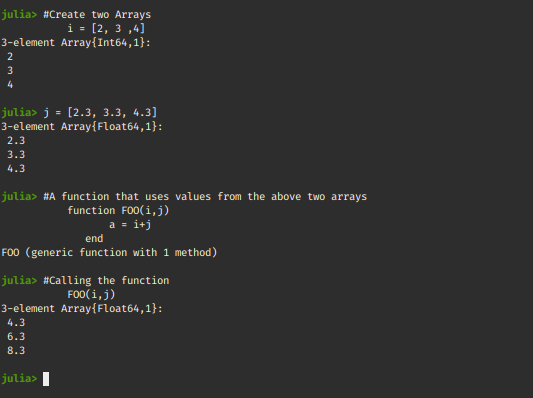• They allow for greater flexibility to represent data.
• Instead of creating different arrays for a different types, they provide the space-efficient way to store all types in one array.
• They provide a unique data type “Any“that allows all sorts of Data Types.

Disavantages of Cell Arrays:

• They are very slow. Julia is a strictly types language(means tha the types are pre-defined)
• We lose information about the data, eg: When we push a String value into a Float array to convert it to a Cell Array, it changes from Array{Float64} to Array{Any} type, hence the information about data types in the Cell Array is lost.

Don’t stop now and take your learning to the next level. Learn all the important concepts of Data Structures and Algorithms with the help of the most trusted course: DSA Self Paced. Become industry ready at a student-friendly price.

My Personal Notes arrow_drop_upCheck out this Author's contributed articles.

If you like GeeksforGeeks and would like to contribute, you can also write an article using contribute.geeksforgeeks.org or mail your article to contribute@geeksforgeeks.org. See your article appearing on the GeeksforGeeks main page and help other Geeks.

Please Improve this article if you find anything incorrect by clicking on the "Improve Article" button below.

Article Tags :
Practice Tags :

Be the First to upvote.

Please write to us at contribute@geeksforgeeks.org to report any issue with the above content.